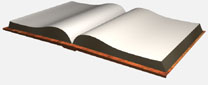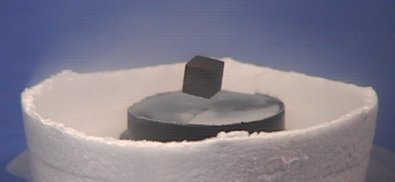# SuperconductorsSuperconductors are materials that demonstrate no resistance to the flow of electric current. That's zero electrical resistance. Therefore, an electric current initiated inside a perfect superconductor will not dissipate with time and will flow forever.

The critical temperature of superconductors is usually given in degrees Kelvin. So what is degrees Kelvin and why do we use this temperature scale? Kelvin is named after the individual Lord Kelvin, who suggested that absolute zero become the base of a new temperature scale. This temperature scale has been adopted by science because it is much easier to work with positive numbers in equations rather than negative numbers encountered in the Celsius and Fahrenheit scales. For instance liquid nitrogen condenses into a liquid at 77 degrees Kelvin. This temperature is equivalent to –196 degrees Celsius or -320 degrees Fahrenheit.

Conversion between the temperature scales is accomplished using a few simple formulas.

To convert degrees Kelvin to Celsius subtract 273. So 100 K is equivalent to –173 C.

To convert Fahrenheit to Celsius

F = 9/5 C + 32

To Convert Celsius to Fahrenheit

C = 5/9 F – 32

Next Page